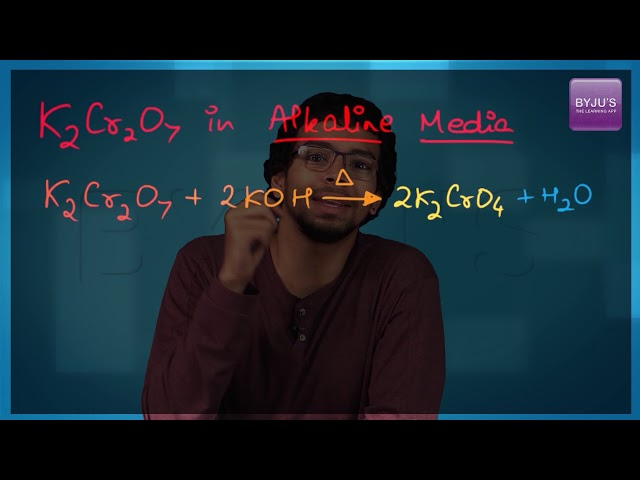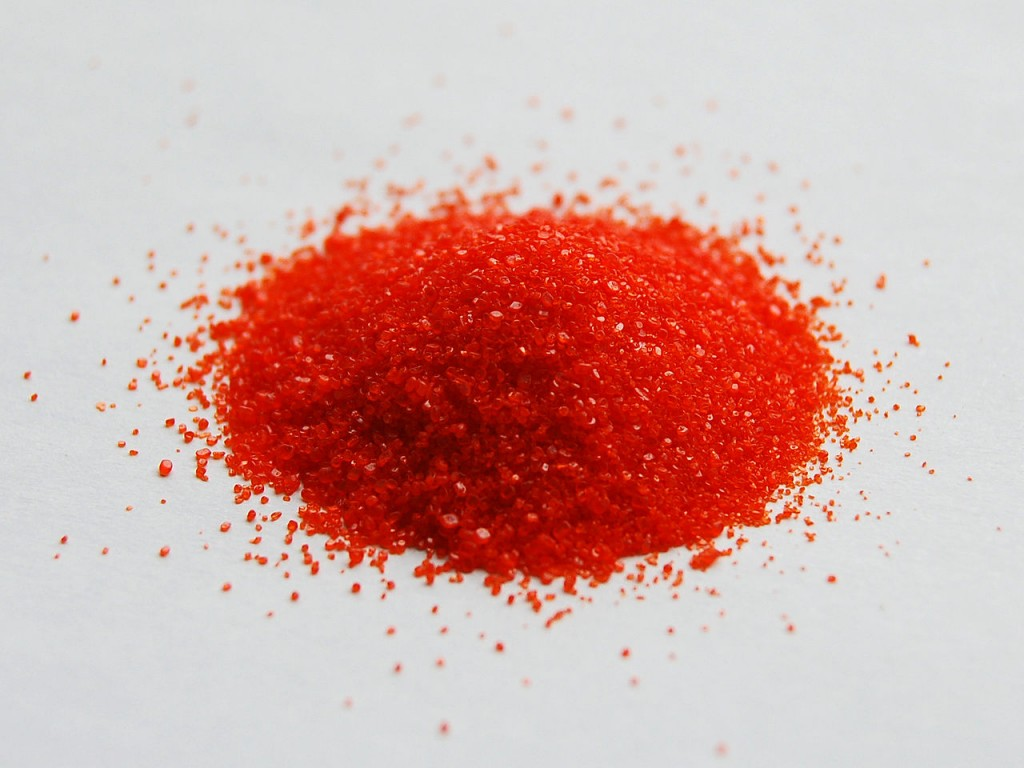# Preparation of Potassium Dichromate and Potassium Permanganate

## What is Potassium Dichromate?

Potassium dichromate or anhydrochromate is prepared by adding to the neutral yellow chromate of potassium in solution, a moderate quantity of one of the stronger acids. Potassium permanganate is commercially prepared by mixing solution of potassium hydroxide and powdered manganese oxide with oxidising agents like potassium chlorate.

## Recommended Videos## Preparation of Potassium Dichromate – K2Cr2O7

• Potassium dichromate is an important chemical used in industries as an oxidizing agent and for the preparation of many other compounds.
• Dichromates are usually prepared from chromates and this is obtained by the combination of chromite ore with sodium/potassium carbonate in the presence of air.Potassium Dichromate

The reaction can be given as:

4FeCr2O4 + 8Na2CO3 + 7O2 $\rightarrow$ 8Na2CrO4 + 2Fe2O3 + 8CO2

The solution of sodium chromate (Na2CrO4) is further purified with sulphuric acid to form a solution from which the crystals of orange coloured sodium dichromate (Na2Cr2O7.2H2O) can be extracted.

2Na2CrO4 + 2H+  $\rightarrow$  Na2Cr2O7 + 2Na+ + H2O

Now potassium dichromate can be obtained by reacting a solution of sodium dichromate with potassium chloride.

Na2Cr2O7 + 2KCl $\rightarrow$  K2Cr2O7 + 2NaCl

Thus we finally obtain the orange crystals of potassium dichromate.

## Preparation of Potassium Permanganate – KMnO4

We can get this (KMnO4) by reacting MnO2 with an alkali metal hydroxide and KNO(oxidizing agent).

This will result in the production of dark green K2MnO4 which disproportionate in an acidic or neutral medium to give permanganate.

2MnO2 + 4KOH + O2 $\rightarrow$  2K2MnO4 + 2H2O

3MnO42- + 4H+ $\rightarrow$  2MnO4 + MnO2 + 2H2O

Therefore, preparation of potassium permanganate involves a reaction of MnO2 with KOH to give MnO42- followed by electrolytic oxidation of manganate to give permanganate ion, MnO4.

After this manganese ion salt gets oxidized by peroxodisulphate to permanganate ion as per the reaction given below.

2Mn2+ + 5S2O82- + 8H2O $\rightarrow$  2MnO4  +10SO42- + 16H+

Thus the dark purple coloured crystals of potassium permanganate are obtained.

2KMnO4 $\rightarrow$  K2MnO4 + MnO2 + O2

## Properties of K2Cr2O7 & KMnO4

### 1. Potassium Dichromate, K2Cr2O7

• On heating potassium dichromate decomposes to form potassium chromate, chromic oxide and oxygen.

4K2Cr2O7 $\rightarrow$  4K2CrO4 + 2CrO3 + 3O2

• It is a powerful oxidizing agent. It oxidizes iodide to iodine.

Cr2O72- + 14H+ + 6I $\rightarrow$  2Cr3+ + 7H2O + 3I2

• The compound has bright red crystals and is used for dyeing, staining, and tanning etc.
• For a medical purpose, it can be an astringent, antiseptic and caustic. It emits toxic chromium fumes when heated
• It is highly corrosive and is a strong oxidizing agent for which, it is used in wood preservatives, pigments manufacture and photochemical processes.

### 2. Potassium permanganate, KMnO4

• When potassium permanganate is heated it gives potassium manganate, manganese dioxide and oxygen.

2KMnO4 $\rightarrow$   K2MnO4 + MnO2 + O2

• It is a powerful oxidizing agent in acidic or alkaline solution.
• It is in the form of purple crystals and gets soluble in hot water
• It is noncombustible but works as a catalyst in the burning of combustible materials.
• It will start a fire when mixed with glycerine.
• It can become toxic in high concentration

## FAQs

1. What is the use of potassium dichromate?
Ans: It is used in many applications as an oxidizing agent and is also used in the preparation of different products such as waxes, paints, glues, etc. Potassium dichromate is carcinogenic and highly toxic as a compound of hexavalent chromium.

2. What does the potassium dichromate test for?
Ans: For organic chemistry, potassium dichromate is an oxidizing agent that is milder than potassium permanganate. It is used for the oxidation of alcohol. This converts primary alcohols into aldehydes and carboxylic acids under more pressing conditions.

3. Is potassium dichromate light-sensitive?
Ans: Clear, light-sensitive orange crystals. Potassium dichromate is used in cotton dyeing as chromium mordant. In black and white image processing, potassium dichromate is used as an intensifier.

4. What is the charge of potassium dichromate?
Ans: K2Cr2O7 is the molecular formula. A reddish-brown color as a solid and a molecular weight of 294.18 grams per mole are the physical properties of potassium dichromate. Potassium dichromate is also referred to as a compound of hexavalent chromium, and chromium oxidation is 6+.

5. Why KMnO4 is a self indicator?
Ans: So the solution loses its pink color once all the permanganate ions are used up in the reaction. It means the end of the reaction and therefore a self-indicator is called potassium permanganate as it acts as an indicator apart from being one of the reactants.